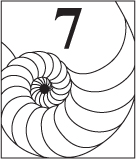### GIBONACCI NUMBERS

We can study properties shared by Fibonacci and Lucas numbers by investigating a number sequence that satisfies the Fibonacci recurrence, but with arbitrary initial conditions. This is our focus in this chapter.

## 7.1 GIBONACCI NUMBERS

Consider the sequence, where, and. The ensuing sequenceis the gibonacci sequence (generalized Fibonacci sequence);is the nth gibonacci number. (A.T. Benjamin and J.J. Quinn coined the term gibonacci .)

Take a good look at the coefficients of a and b in the various terms of this sequence. They follow the same pattern we saw in Chapter 5 for powers of: The coefficients of a and b are Fibonacci numbers. We will now pinpoint these two coefficients in the following theorem.

Get Fibonacci and Lucas Numbers with Applications, Volume 1, 2nd Edition now with the O’Reilly learning platform.

O’Reilly members experience books, live events, courses curated by job role, and more from O’Reilly and nearly 200 top publishers.